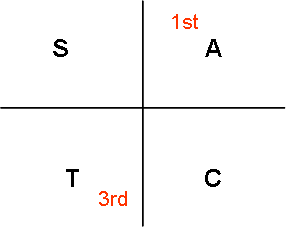### Basic Trigonometry

Basic Angles / Reference angles

Using your calculator, determine what is sin 30°, sin 150°, sin 210° and sin 330°?

sin 30° = 0.5
sin 150° = 0.5
sin 210°= -0.5
sin 330°=-0.5

What do you think is the relationship between these angles ?

30° = 30° (1st quadrant )
150° = 180° – 30° (2nd quadrant)
210° = 180° + 30° (3rd quadrant)
330° = 360° – 330° (4th quadrant)

You would realise that sine is positive in the 1st and 2nd qudrants

You can carry out the same exercise with cos and tan
cosine is positive in the 1st and 4th quadrant
tangent is positive in the 1st and 3rd quadrantWe can use the diagram above to help us remember which functions are positive in the quadrants

A means all the functions are positive in 1st quadrant
T means that only tangent is positive in the 3rd quadrant

I remember this as All Science Teachers are CharmingExample 1
Given thatfor x betweenandDetermine the value of
a) sin x
b) sin 2x

Given that the side adjacent to angle x is 5 units and the hypotenuse is 13 units

we can work out the side opposite angle x using Pythagoras theorem

The side opposite x is 12 units

a)note that sin x in the second quadrant is positive

b)Example 2

find the following:

Given that the side opposite to angle x is 4 units and the hypotenuse is 5 units

we can work out the side adjacent to angle x using Pythagoras theorem

The side adjacent to angle x is 3 units

note that cos x in the second quadrant is negative

a)

b)
Making use of the identity

c)

Example 3

Note that this question is probably too tedious for the O Level exam. In the actual exams, you will probably be asked what is

Basic angles form the foundation of solving trigo equations.

What's Next ?

1.I am very happy to locate your website. I just wanted to thank you for the time you spent on this great article. I definitely enjoyed reading it and I have you bookmarked to check out new stuff you post.

Yong
www.gofastek.com

2.I liked your work and, as a result, the manner you presented this content about High School Math Tutor.It is a valuable paper for us. Thank you for sharing this blog with us.

3.A very delightful article that you have shared here. Your blog is a valuable and engaging article for us, and also I will share it with my companions who need this info. Thankful to you for sharing an article like this. Singapore Mathematics

4.It's a nice article, Which you have shared here about the Your article is very informative and I really liked the way you expressed your views in this post. Thank you.Celpip Classes in Vancouver